Courses

# Test: Power Systems- 3

## 10 Questions MCQ Test RRB JE for Electrical Engineering | Test: Power Systems- 3

Description
This mock test of Test: Power Systems- 3 for Electrical Engineering (EE) helps you for every Electrical Engineering (EE) entrance exam. This contains 10 Multiple Choice Questions for Electrical Engineering (EE) Test: Power Systems- 3 (mcq) to study with solutions a complete question bank. The solved questions answers in this Test: Power Systems- 3 quiz give you a good mix of easy questions and tough questions. Electrical Engineering (EE) students definitely take this Test: Power Systems- 3 exercise for a better result in the exam. You can find other Test: Power Systems- 3 extra questions, long questions & short questions for Electrical Engineering (EE) on EduRev as well by searching above.
QUESTION: 1

### A single core cable has a conductor diameter of 3 cm and an overall diameter of 6 cm. If the resistivity of the insulating material is 9 × 108 MΩ/cm while the length of the cable is 3 km then calculate the Insulation Resistance of this cable.

Solution:

We known that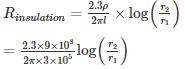=1098.73 log 2 = 330.74 MΩ

*Answer can only contain numeric values
QUESTION: 2

### An interconnected cable having a reactance of j0.2 pu links two generating stations G1 and G2 as shown below. If |V1| = |V2| = 1 pu, the reactive power demand by transmission line is ______ (in pu)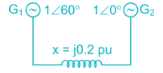Solution: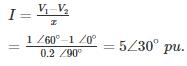Reactive power demand by transmission line

= |I2| X

= (5)2 × 0.2

= 5 pu

QUESTION: 3

### A three-phase overhead transmission line has its conductors horizontally spaced with spacing between adjacent conductors to ‘d’. If now the conductors of the line are rearranged to form an equilateral triangle of sides equal to d then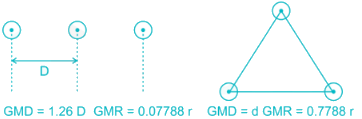Solution:

Since GMR and r same in both cases L/ph proposal to Ln (G.M.D) and C/ph proposal to 1/Ln (GMD)

∴ Capacitance will increase and inductance will decrease

*Answer can only contain numeric values
QUESTION: 4

The string efficiency of a string insulator with the bottom unit having a 50 % of the total voltage is 40%. The number of units in the string insulator is____

Solution: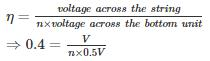⇒ n = 5

*Answer can only contain numeric values
QUESTION: 5

An electric train taking of constant current of 800A moves on a section of line between two substations 10 km apart maintained at 600 and 640 V respectively the track resistance is 0.05 Ω/km both go and return. The point of minimum potential along the track is at a distance from 640 V substation is – (in km)

Solution:Potential of P, Vp = 600 – I1 (0.05)x

= 640 – (800 – I1) (0.05) (10 – x)

⇒ (800 – I1) (0.05) (10 – x) = 40 + I1 (0.05) x

⇒ (800 – I1) (10 – x) = 800 + I1 x

⇒ 800 + I1 x  – 10I1 – 800x = 800 + I1 x

⇒ 100I1 + 800x = 7200

⇒ I1 = 720 – 80 x

Now, Vp = 600 – (720 – 80x) 0.05 x

= 600 – 360x + 4x2

For Vp to be minimum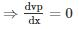⇒ -36 + 8x = 0

⇒ x = 4.5 km

Distance from 640V substation is = 10 – x = 5.5 km

*Answer can only contain numeric values
QUESTION: 6

A 50 km long cable has a capacitance of 0.2 μF/km between any two conductors. When the other conductor is connected to sheath. The supply voltage is 22 kV, 50 Hz, 3 – ϕ. The charging current IPh is________ (in A)

Solution:

L = 50 km

Ceq = 0.2 μF / km

cph=2×Ceqcph=2×Ceq

= 0.4 μF / km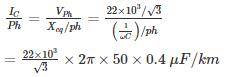= 1.596 A / km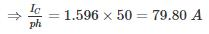QUESTION: 7

In a 5 insulator disc, string capacitance between each unit and earth is 1/6th of the mutual capacitance. The voltage distribution across the middle unit as % of the voltage applied is –

Solution: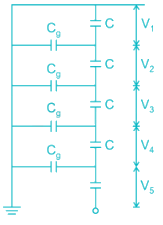Given that,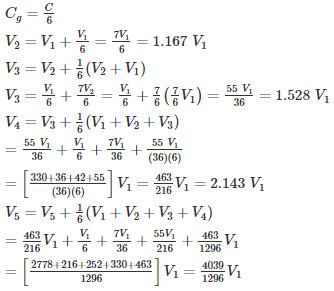= 3.12 V1

V= V1 + V2 + V3 + V4 + V5 = 8.95

V3 in percentage of V is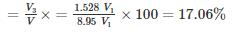*Answer can only contain numeric values
QUESTION: 8

A 3 phase line has conductors 1 cm in diameter spaced equilaterally 2 m apart. The dielectric strength of air is 30 kV (max) per cm. Air density factor is taken as 0.9 and irregularity factor is 0.85. The disruptive critical voltage (rms), for the line is ________ (in kV)

Solution:

Given that,

Diameter of conductor (D) = 1 cm

Distance between conductors (d) = 2m

Dielectric strength of air (gmax) = 30 kV/cm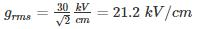Air density factor (δ) = 0.9

Irregularity factor (m) = 0.85

The critical disruptive voltage is given by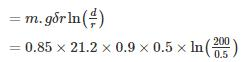= 48.585 kV/phase.

Disruptive voltage for the line = 48.585 × √3 = 84.15 kV

QUESTION: 9

The capacitance of a 3-core lead sheathed cable measured between any two of the conductors with sheath earthed is 0.3 μF per km. Determine the equivalent star connected capacity and the MVA required to keep 20 km of the cable charged when connected to 25 kV, 50 Hz supply

Solution:

The equivalent circuit is shown in fig.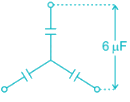Given that, capacitance is 0.3 μF/km

For 20 km cable, capacitance is 0.3 × 20 = 6 μF

Since, the capacitance measured is 6 μF between the conductors, the capacitance per phase = 2 × 6 = 12 μF

3-phase MVA required

= V2ωc = (25 × 1 03) 2× 2π × 50 × 12 × 10−6

= 2.356 MVA

*Answer can only contain numeric values
QUESTION: 10

A 3 phase line operating at 150 kV which has a conductor of 1.5 cm diameter arranged in a 4-meter delta. Assume air density factor of 1.2 and the dielectric strength of air to be 21kVcm21kVcm. Calculate the corona loss ____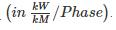Solution: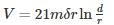= 21 × 1.2 × 1 × 0.75 ln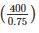Line to line voltage is = 205.55 kV

Since the operating voltage is 150 kV, the corona loss will be absent.

⇒ Corona loss = 0.